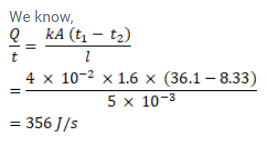Deepak Scored 45->99%ile with Bounce Back Crack Course. You can do it too!

# The normal body - temperature of a person

Question:

The normal body - temperature of a person is $97^{\circ} \mathrm{F}$ Calculate the rate at which heat is flowing out of his body through the clothes assuming the following values. Room temperature $=47^{\circ} \mathrm{F}$ surface of the body under clothes $=1.6 \mathrm{~m}^{2}$ conductivity of the cloth $=0.04 \mathrm{~J} / \mathrm{m}-\mathrm{s}^{\circ} \mathrm{C}$, thickness of the cloth $=0.5 \mathrm{~cm}$.

Solution: